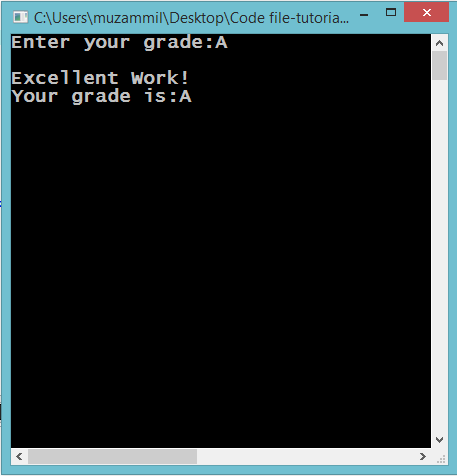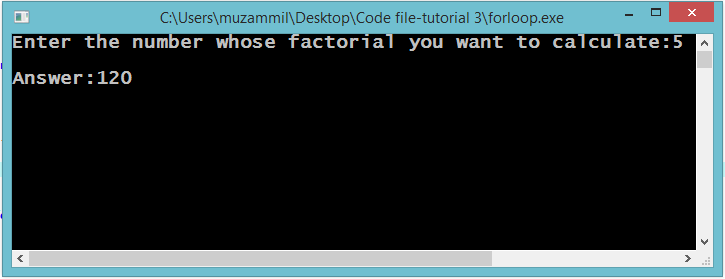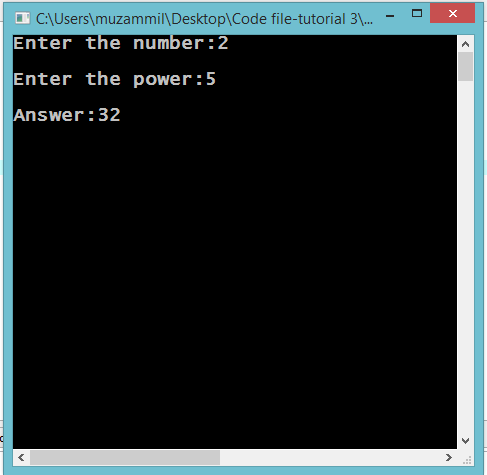# Switch Statement and Loops

## Switch Statements and Loops

In this part you will learn: 1. C syntax 2. Switch Statement 3. For Loop In this tutorial I will teach you about Switch statements and some basics of For loop. The first program is related to switch statement. The second and third program is about for loop. Switch Statement Basic Step: Open Dev C++ then File > new > source file and start writing the code below.
`#include<stdio.h>#include<conio.h>`
These two are the most common and basic header files. The first one stdio.h is used to grant us most of the basic functions of C like Input and Output functions. The second one conio.h provides us functions like getch() which is used to pause our screen until we press a button.
`int main(){	char grade;	printf("Enter your grade:");	scanf("%c",&grade);	switch(grade)	{		case 'A':			printf("\nExcellent Work!");			break;		case 'B':			printf("\nWelldone!");			break;		case 'C':			printf("\nNice Work!");			break;		case 'D':			printf("\nYou Passed!");			break;		case 'F':			printf("\nFail");			break; 		default:			printf("\nInvalid grade.");	}	printf("\nYour grade is:%c",grade);		getch();}`
This program takes input a grade and outputs a remark according to that certain grade. First of all we have declared a character variable. Then we have taken input from the user in that character variable. Then comes are switch statement. Let me tell you that a switch statement almost works in a same way like if else conditional statement. We pass switch function our variable and then using cases we check it according to our requirements. If the variable does not meet any case it executes the default part which is just like the else part. We place break key word after every statement because we do not want switch to check any other case after meeting the correct value of certain case. So if our character matches any of the case the printf() statement corresponding to that statement will b executed and the switch will break and the program will be pause at getch() function. Otherwise the default statement will be executed and the program will still stop at getch() function. Execute > compile then Execute > run OutputFor Loop Basic Step: Open Dev C++ then File > new > source file and start writing the code below.
`#include<stdio.h>#include<conio.h>`
These two are the most common and basic header files. The first one stdio.h is used to grant us most of the basic functions of C like Input and Output functions. The second one conio.h provides us functions like getch() which is used to pause our screen until we press a button. There are three type of loops in C. 1. For loop 2. While loop 3. Do While loop In this tutorial we will only cover for loop. This is a program that asks the user a number and calculate its factorial.
`int main(){		int fact=1,n;	printf("Enter the number whose factorial you want to calculate:");	scanf("%d",&n);  	for(int i=n;i>0;i--)	{		fact=fact*i; 	}	printf("\nAnswer:%d",fact); 	getch();}`
In C loop statement allows us to execute a statement or a group of statement multiple time. The above program shows a For loop. In For loop we write three Statements. There are three parts in a for loop. 1. Initialization 2. Condition 3. Increment The first statement is initialization. This is initializing the loop counter. It is mostly set ot 0 or 1. But in our case we our initializing it with n. Then comes the condition. This condition is for the counter. The counter keeps on executing the loop till this condition is met. Then when the condition becomes false for the counter it stops executing the loop. Then at last there is increment. This statement keeps on incrementing in the counter on every iteration. In this program we are calculating the factorial so as you know when we calculate a factorial we multiply every number from 1 to that number whose factorial we want to calculate. In this code we are moving in backward direction. From multiplying ‘n’ with every number all the way to n. Our number keeps on accumulating in integer variable fact. And at the end we output our answer. Execute > compile then Execute > run OutputCalculating Power Basic Step: Open Dev C++ then File > new > source file and start writing the code below.
`#include<stdio.h>#include<conio.h>`
These two are the most common and basic header files. The first one stdio.h is used to grant us most of the basic functions of C like Input and Output functions. The second one conio.h provides us functions like getch() which is used to pause our screen until we press a button. In this program we will calculate the power of a number. Both the value will be entered by the user.
`#include<stdio.h>#include<conio.h> int main(){	int n,p,product=1;	printf("Enter the number:");	scanf("%d",&n);	printf("\nEnter the power:");	scanf("%d",&p);  	for(int i=0;i<p;i++)	{		product=product*n;	} 	printf("\nAnswer:%d",product); 	getch();}`
In this program we took input from the user about the number and its power. Then we started the loop keeping the counter i=0. And we multiplied the number power times with itself. In this way we calculated the power using for loop and accumulated the answer in product variable. And then displayed the answer. Execute > compile then Execute > run Output